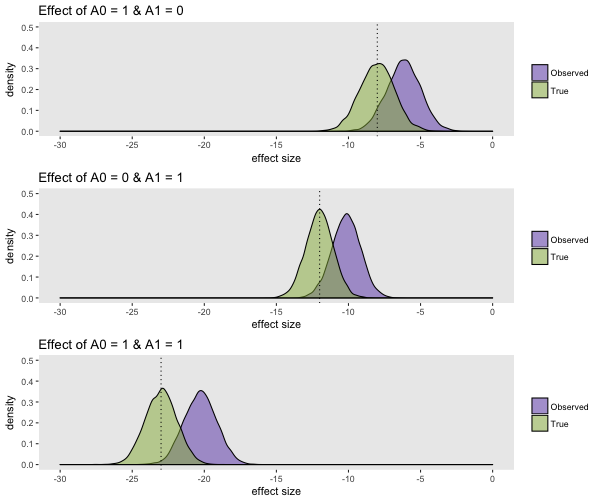# When there's a fork in the road, take it. Or, taking a look at marginal structural models.

I am going to cut right to the chase, since this is the third of three posts related to confounding and weighting, and it’s kind of a long one. (If you want to catch up, the first two are here and here.) My aim with these three posts is to provide a basic explanation of the marginal structural model (MSM) and how we should interpret the estimates. This is obviously a very rich topic with a vast literature, so if you remain interested in the topic, I recommend checking out this (as of yet unpublished) text book by Hernán & Robins for starters.

The DAG below is a simple version of how things can get complicated very fast if we have sequential treatments or exposures that both affect and are affected by intermediate factors or conditions.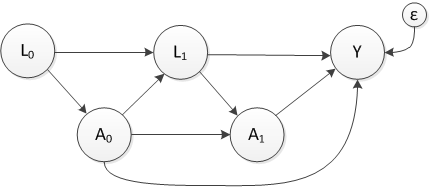$$A_0$$ and $$A_1$$ represent two treatment points and $$L_0$$ and $$L_1$$ represent measurements taken before and after treatments, respectively. Both treatments and at least $$L_1$$ affect outcome $$Y$$. (I am assuming that the $$A$$’s and $$L$$’s are binary and that $$Y$$ is continuous. $$\epsilon$$ is $$N(0, \sigma_\epsilon^2)$$.)

An example of this might be a situation where we are interested in the effect of a drug treatment on mental health well-being for patients with prehypertension or hypertension. A physician’s decision to administer the drug at each visit is influenced by the patient’s level of hypertension. In turn, the treatment $$A_0$$ potentially reduces the probability of hypertension - $$P(L_1=1)$$. And finally, $$L_1$$ influences the next treatment decision and ultimately the mental health outcome $$Y$$.

The complicating factor is that the hypertension level following the first treatment ($$L_1$$) is both a mediator the effect of treatment $$A_0$$ and confounder of the treatment effect $$A_1$$ on $$Y$$. To get an unbiased estimate the effect of the combined treatment regime ($$A_0$$ and $$A_1$$) we need to both control for $$L_1$$ and not control for $$L_1$$. This is where MSMs and inverse probability weighting (IPW) come into play.

The MSM is marginal in the sense that we’ve been talking about in this series - the estimate will be a population-wide estimate that reflects the mixture of the covariates that influence the treatments and outcomes (in this case, the $$L$$’s). It is structural in the sense that we are modeling potential outcomes. Nothing has changed from the last post except for the fact that we are now defining the exposures as a sequence of different treatments (here $$A_0$$ and $$A_1$$, but could easily extend to $$n$$ treatments - up to $$A_n$$.)

### Imagine an experiment …

To understand the MSM, it is actually helpful to think about how a single individual fits into the picture. The tree diagram below literally shows that. The MSM posits a weird experiment where measurements (of $$L$$) are collected and treatments ($$A$$) are assigned repeatedly until a final outcome is measured. In this experiment, the patient is not just assigned to one treatment arm, but to both! Impossible of course, but that is the world of potential outcomes.

At the start of the experiment, a measurement of $$L_0$$ is collected. This sends the patient down the one of the branches of the tree. Since the patient is assigned to both $$A_0=0$$ and $$A_0=1$$, she actually heads down two different branches simultaneously. Following the completion of the first treatment period $$A_0$$, the second measurement ($$L_1$$) is collected. But, two measurements are taken for the patient - one for each branch. The results need not be the same. In fact, if the treatment has an individual-level effect on $$L_1$$, then the results will be different for this patient. In the example below, this is indeed the case. Following each of those measurements (in parallel universes), the patient is sent down the next treatment branches ($$A_1$$). At this point, the patient finds herself in four branches. At the end of each, the measurement of $$Y$$ is taken, and we have four potential outcomes for individual {i}: $$Y^i_{00}$$, $$Y^i_{10}$$, $$Y^i_{01}$$, and $$Y^i_{11}$$.

A different patient will head down different branches based on his own values of $$L_0$$ and $$L_1$$, and will thus end up with different potential outcomes. (Note: the values represented in the figure are intended to be average values for that particular path.)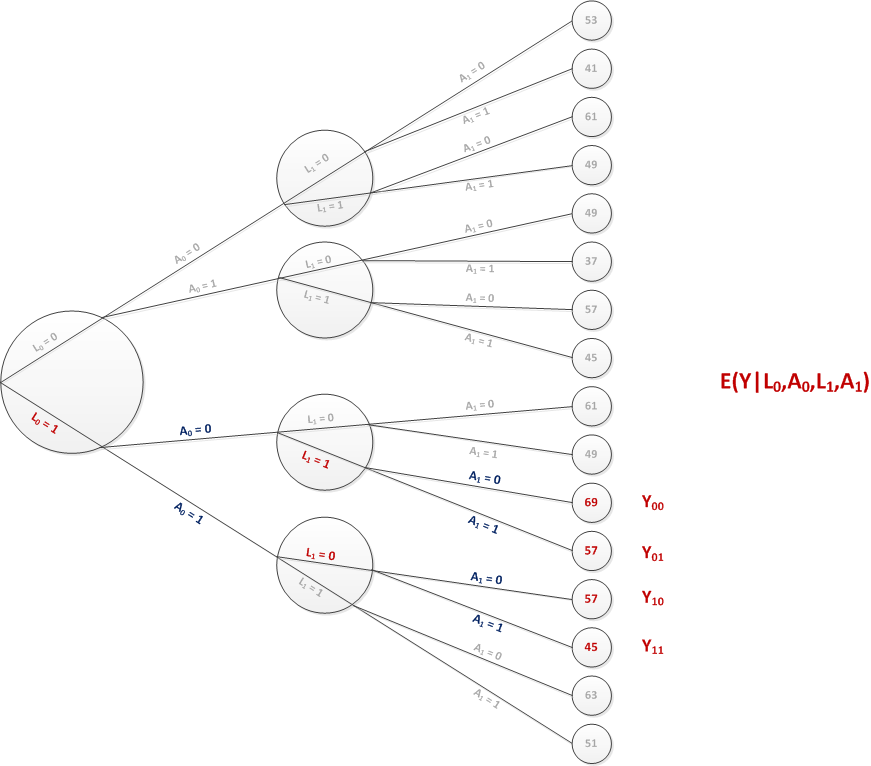### How do we define the causal effect?

With four potential outcomes rather than two, it is less obvious how to define the causal effect. We could, for example, consider three separate causal effects by comparing each of the treatment “regimes” that include at least one exposure to the intervention to the single regime that leaves the patient entirely unexposed. That is, we could be interested in (at the individual $$i$$ level) $$E^i_1 = Y^i_{10}-Y^i_{00}$$, $$E^i_2 = Y^i_{01}-Y^i_{00}$$, and $$E^i_3 = Y^i_{11}-Y^i_{00}$$. This is just one possibility; the effects of interest are driven entirely by the research question.

When we have three or four or more intervention periods, the potential outcomes can start to pile up rapidly (we will have $$2^n$$ potential outcomes for a sequence of $$n$$ treatments.) So, the researcher might want to be judicious in deciding which contrasts to be made. Maybe something like $$Y_{1111} - Y_{0000}$$, $$Y_{0111} - Y_{0000}$$, $$Y_{0011} - Y_{0000}$$, and $$Y_{0001} - Y_{0000}$$ for a four-period intervention. This would allow us to consider the effect of starting (and never stopping) the intervention in each period compared to never starting the intervention at all. By doing this, though, we would be using only 5 out of the 16 potential outcomes. If the remaining 11 paths are not so rare, we might be ignoring a lot of data.

### The marginal effect

The tree below represents an aggregate set of branches for a sample of 5000 individuals. The sample is initially characterized only by the distribution of $$L_0$$. Each individual will go down her own set of four paths, which depend on the starting value of $$L_0$$ and how each value of $$L_1$$ responds in the context of each treatment arm.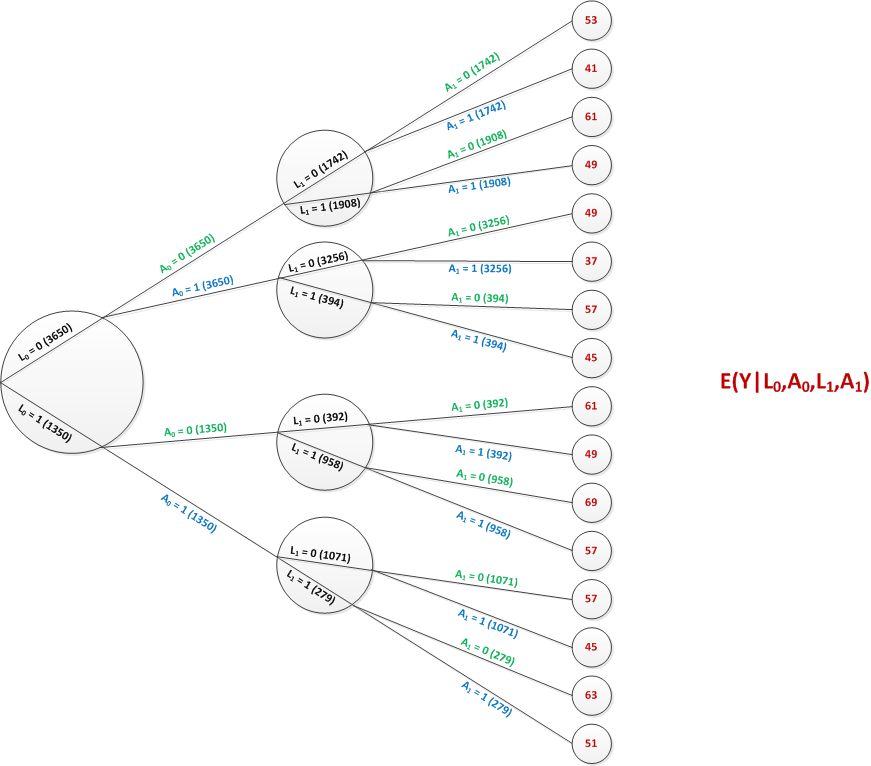Each individual $$i$$ (at least in theory) has four potential outcomes: $$Y^i_{00}$$, $$Y^i_{10}$$, $$Y^i_{01}$$, and $$Y^i_{11}$$. Averaging across the sample provides a marginal estimate of each of these potential outcomes. For example, $$E(Y_{00})=\sum_i{Y^i_{00}}/5000$$. This can be calculated from the tree as $(1742*53 + 1908*61 + 392*61 + 958*69)/5000 = 59.7$ Similarly, $$E(Y_{11}) = 40.1$$ The sample average causal effects are estimated using the respective averages of the potential outcomes. For example, $$E_3$$ at the sample level would be defined as $$E(Y_{11}) - E(Y_{00}) = 40.1 - 59.7 = -19.6$$.

### Back in the real world

In reality, there are no parallel universes. Maybe we could come up with an actual randomized experiment to mimic this, but it may be difficult. More likely, we’ll have observed data that looks like this: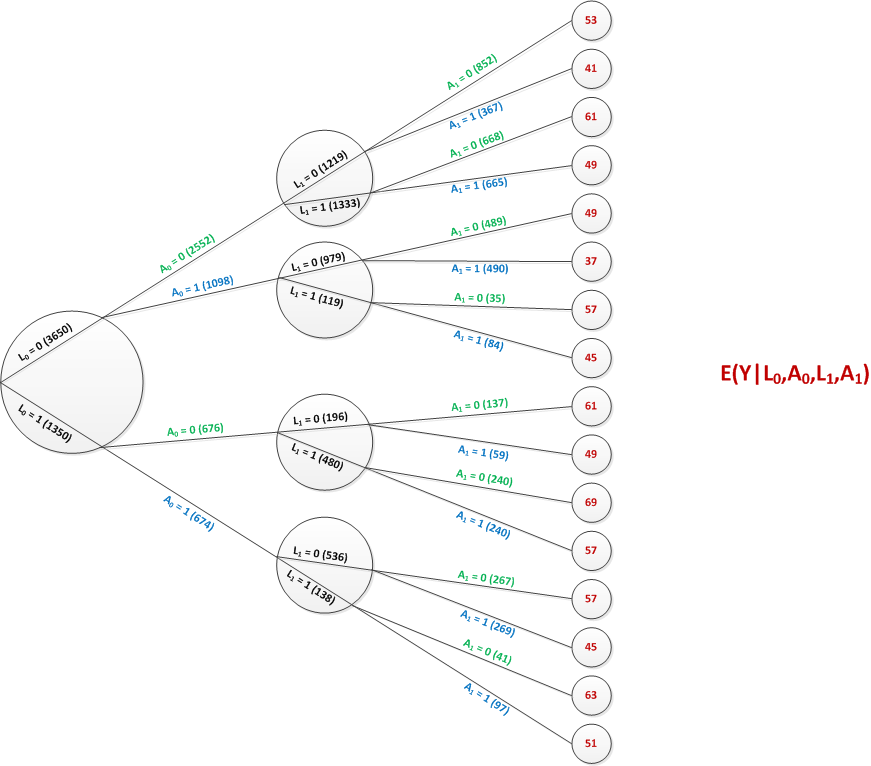Each individual heads down his or her own path, receiving a single treatment at each time point. Since this is not a randomized trial, the probability of treatment is different across different levels of $$L_0$$ and $$L_1$$ and that $$L_0$$ and $$L_1$$ are associated with the outcome (i.e. there is confounding).

### Estimating the marginal effects

In the previous posts in this series, I provided some insight as to how we might justify using observed data only to estimate these sample-wide average potential outcomes. The most important assumption is that when we have measured all confounders, we may be able to say, for example, $$E(Y_{01}) = E(Y | A_0 = 0 \ \& \ A_1 = 1 )$$. The potential outcome for everyone in the sample is equal to the observed outcome for the subgroup who actually followed the particular path that represents that potential outcome. We will make the same assumption here.

At the start of this post, I argued that given the complex nature of the data generating process (in particular given that $$L_1$$ is both a mediator and confounder), it is challenging to get unbiased estimates of the intervention effects. One way to do this with marginal structural models (another way is using g-computation, but I won’t talk about that here). Inverse probability weighting converts the observed tree graph from the real world to the marginal tree graph so that we can estimate sample-wide average (marginal) potential outcomes as an estimate for some population causal effects.

In this case, the inverse probability weight is calculated as $IPW = \frac{1}{P(A_0=a_0 | L_0=l_0) \times P(A_1=a_1 | L_0=l_0, A_0=a_0, L_1=l_1)}$ In practice, we estimate both probabilities using logistic regression or some other modeling technique. But here, we can read the probabilities off the tree graph. For example, if we are interested in the weight associated with individuals observed with $$L_0=1, A_0=0, L_1=0, \textbf{and } A_1=1$$, the probabilities are $P(A_0 = 0 | L_0=1) = \frac{676}{1350}=0.50$ and $P(A_1=1 | L_0=1, A_0=0, L_1=0) = \frac{59}{196} = 0.30$

So, the inverse probability weight for these individuals is $IPW = \frac{1}{0.50 \times 0.30} = 6.67$ For the 59 individuals that followed this pathway, the weighted number is $$59 \times 6.67 = 393$$. In the marginal world of parallel universes, there were 394 individuals.

### Simulating data from an MSM

Before I jump into the simulation, I do want to reference a paper by Havercroft and Didelez that describes in great detail how to generate data from a MSM with time-dependent confounding. It turns out that the data can’t be generated exactly using the intial DAG (presented above), but rather needs to come from something like this: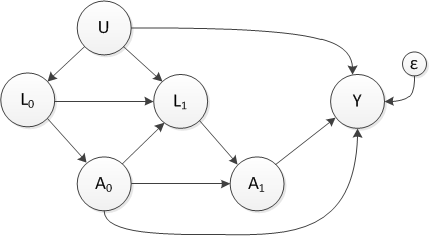where $$U$$ is an unmeasured, maybe latent, covariate. The observed data (that ignores $$U$$) will indeed have a DAG that looks like the one that we started with.

When doing simulations with potential outcomes, we can generate all the potential outcomes for each individual using a parallel universe approach. The observed data (treatment choices and observed outcomes) are generated separately. The advantage of this is that we can confirm the true causal effects because we have actually generated potential outcomes. The disadvantage is that the code is considerably more complicated and the quantity of data generated grows. The situation is not so bad with just two treatment periods, but the size of the data increases exponentially with the number of treatments: as I mentioned earlier, there will be $$2^n$$ potential outcomes for each individual.

Alternatively, we can just generate the observed data directly. Since we know the true causal parameters we actually “know” the causal effects and can compare our estimates.

I will go through the convoluted approach because I think it clarifies (at least a bit) what is going on. As an addendum, I will show how all of this could be done in a few lines of code if we take the second approach …

library(broom)
library(simstudy)

# define U, e and L0

defA0 <- defData(varname = "U", formula = "0;1", dist = "uniform")
defA0 <- defData(defA0, varname = "e", formula = 0,
variance = 4, dist = "normal")
defA0<- defData(defA0, varname = "L0", formula = "-2.66+ 3*U",
dist = "binary", link = "logit")

# generate the data

set.seed(1234)
dtA0 <- genData(n = 50000, defA0)
dtA0[1:6]
##    id         U          e L0
## 1:  1 0.1137034 -3.5951796  0
## 2:  2 0.6222994 -0.5389197  0
## 3:  3 0.6092747  1.0675660  0
## 4:  4 0.6233794 -0.7226909  1
## 5:  5 0.8609154  0.8280401  0
## 6:  6 0.6403106  3.3532399  0

Now we need to create the two parallel universes - assigning each individual to both treatments. simstudy has a function addPeriods to generate longitudinal data. I am not doing that here, but can generate 2-period data and change the name of the “period” field to “A0”.

dtA0 <- addPeriods(dtA0, 2)
setnames(dtA0, "period", "A0")
dtA0[1:6]
##    id A0         U          e L0 timeID
## 1:  1  0 0.1137034 -3.5951796  0      1
## 2:  1  1 0.1137034 -3.5951796  0      2
## 3:  2  0 0.6222994 -0.5389197  0      3
## 4:  2  1 0.6222994 -0.5389197  0      4
## 5:  3  0 0.6092747  1.0675660  0      5
## 6:  3  1 0.6092747  1.0675660  0      6

Now we are ready to randomly assign a value of $$L_1$$. The probability is lower for cases where $$A_0 = 1$$, so individuals themselves may have different values of $$L_1$$ in the alternative paths.

# generate L1 as a function of U, L0, and A0

formula = "-1.2 + 3*U + 0.2*L0 - 2.5*A0",

dtA0[1:6]
##    id A0         U          e L0 timeID L1
## 1:  1  0 0.1137034 -3.5951796  0      1  0
## 2:  1  1 0.1137034 -3.5951796  0      2  0
## 3:  2  0 0.6222994 -0.5389197  0      3  1
## 4:  2  1 0.6222994 -0.5389197  0      4  0
## 5:  3  0 0.6092747  1.0675660  0      5  0
## 6:  3  1 0.6092747  1.0675660  0      6  0
# L1 is clearly a function of A0

dtA0[, .(prob_L1 = mean(L1)), keyby = .(L0,A0)]
##    L0 A0   prob_L1
## 1:  0  0 0.5238369
## 2:  0  1 0.1080039
## 3:  1  0 0.7053957
## 4:  1  1 0.2078551

Now we create two additional parallel universes for treatment $$A_1$$ and the potential outcomes. This will result in four records per individual:

dtA1 <- addPeriods(dtA0, 2)
setnames(dtA1, "period", "A1")

formula = "39.95 + U*40 - A0 * 8 - A1 * 12 + e",
dist = "nonrandom")

dtA1[1:8]
##    id A1 A0         U          e L0 timeID L1     Y_PO
## 1:  1  0  0 0.1137034 -3.5951796  0      1  0 40.90296
## 2:  1  0  1 0.1137034 -3.5951796  0      2  0 32.90296
## 3:  1  1  0 0.1137034 -3.5951796  0      3  0 28.90296
## 4:  1  1  1 0.1137034 -3.5951796  0      4  0 20.90296
## 5:  2  0  0 0.6222994 -0.5389197  0      5  1 64.30306
## 6:  2  0  1 0.6222994 -0.5389197  0      6  0 56.30306
## 7:  2  1  0 0.6222994 -0.5389197  0      7  1 52.30306
## 8:  2  1  1 0.6222994 -0.5389197  0      8  0 44.30306

Not surprisingly, the estimates for the causal effects mirror the parameters we used to generate the $$Y$$’s above …

# estimate for Y_00 is close to what we estimated from the tree

Y_00 <- dtA1[A0 == 0 & A1 == 0, mean(Y_PO)]
Y_00
##  59.96619
Y_10 <- dtA1[A0 == 1 & A1 == 0, mean(Y_PO)]
Y_01 <- dtA1[A0 == 0 & A1 == 1, mean(Y_PO)]
Y_11 <- dtA1[A0 == 1 & A1 == 1, mean(Y_PO)]

# estimate 3 causal effects

c(Y_10 - Y_00,  Y_01 - Y_00, Y_11 - Y_00)
##   -8 -12 -20

Now that we’ve generated the four parallel universes with four potential outcomes per individual, we will generate an observed treatment sequence and measurements of the $$L$$’s and $$Y$$ for each individual. The observed data set will have a single record for each individual:

dt <- dtA1[A0 == 0 & A1 == 0, .(id, L0)]
dt
##           id L0
##     1:     1  0
##     2:     2  0
##     3:     3  0
##     4:     4  1
##     5:     5  0
##    ---
## 49996: 49996  1
## 49997: 49997  0
## 49998: 49998  1
## 49999: 49999  0
## 50000: 50000  1

$$A_0$$ is a function of $$L_0$$:

dtAdd <- defDataAdd(varname = "A0",
formula = "0.3 + L0 * 0.2", dist = "binary" )

dt[, mean(A0), keyby= L0]
##    L0        V1
## 1:  0 0.3015964
## 2:  1 0.4994783

Now, we need to pull the appropriate value of $$L_1$$ from the original data set that includes both possible values for each individual. The value that gets pulled will be based on the observed value of $$A_0$$:

setkeyv(dt, c("id", "A0"))
setkeyv(dtA1, c("id", "A0"))

dt <- merge(dt, dtA1[, .(id, A0, L1, A1) ], by = c("id", "A0"))
dt <- dt[A1 == 0, .(id, L0, A0, L1)]
dt
##           id L0 A0 L1
##     1:     1  0  1  0
##     2:     2  0  1  0
##     3:     3  0  0  0
##     4:     4  1  1  1
##     5:     5  0  0  1
##    ---
## 49996: 49996  1  1  0
## 49997: 49997  0  1  0
## 49998: 49998  1  1  0
## 49999: 49999  0  0  1
## 50000: 50000  1  0  0

Finally, we generate $$A_1$$ based on the observed values of $$A_0$$ and $$L_1$$, and select the appropriate value of $$Y$$:

dtAdd <- defDataAdd(varname = "A1",
formula = "0.3 + L1 * 0.2 + A0 * .2", dist = "binary")

# merge to get potential outcome that matches actual path

setkey(dt, id, L0, A0, L1, A1)
setkey(dtA1, id, L0, A0, L1, A1)
dtObs <- merge(dt, dtA1[,.(id, L0, A0, L1, A1, Y = Y_PO)])

dtObs
##           id L0 A0 L1 A1        Y
##     1:     1  0  1  0  0 32.90296
##     2:     2  0  1  0  1 44.30306
##     3:     3  0  0  0  1 53.38856
##     4:     4  1  1  1  1 44.16249
##     5:     5  0  0  1  0 75.21466
##    ---
## 49996: 49996  1  1  0  0 74.09161
## 49997: 49997  0  1  0  0 50.26162
## 49998: 49998  1  1  0  0 73.29376
## 49999: 49999  0  0  1  0 52.96703
## 50000: 50000  1  0  0  0 57.13109

If we do a crude estimate of the causal effects using the unadjusted observed data, we know we are going to get biased estimates (remember the true causal effects are -8, -12, and -20):

Y_00 <- dtObs[A0 == 0 & A1 == 0, mean(Y)]
Y_10 <- dtObs[A0 == 1 & A1 == 0, mean(Y)]
Y_01 <- dtObs[A0 == 0 & A1 == 1, mean(Y)]
Y_11 <- dtObs[A0 == 1 & A1 == 1, mean(Y)]

c(Y_10 - Y_00,  Y_01 - Y_00, Y_11 - Y_00)
##   -6.272132 -10.091513 -17.208856

This biased result is confirmed using an unadjusted regression model:

lmfit <- lm(Y ~ A0 + A1, data = dtObs)
tidy(lmfit)
##          term   estimate  std.error statistic p.value
## 1 (Intercept)  58.774695 0.07805319 753.00828       0
## 2          A0  -6.681213 0.10968055 -60.91520       0
## 3          A1 -10.397080 0.10544448 -98.60241       0

Now, shouldn’t we do better if we adjust for the confounders? I don’t think so - the parameter estimate for $$A_0$$ should be close to $$8$$; the estimate for $$A_1$$ should be approximately $$12$$, but this is not the case, at least not for both of the estimates:

lmfit <- lm(Y ~ L0 + L1 + A0 + A1, data = dtObs)
tidy(lmfit)
##          term   estimate  std.error  statistic p.value
## 1 (Intercept)  53.250244 0.08782653  606.31157       0
## 2          L0   7.659460 0.10798594   70.93016       0
## 3          L1   8.203983 0.10644683   77.07119       0
## 4          A0  -4.369547 0.11096204  -39.37875       0
## 5          A1 -12.037274 0.09592735 -125.48323       0

Maybe if we just adjust for $$L_0$$ or $$L_1$$?

lmfit <- lm(Y ~ L1 + A0 + A1, data = dtObs)
tidy(lmfit)
##          term   estimate  std.error  statistic       p.value
## 1 (Intercept)  54.247394 0.09095074  596.44808  0.000000e+00
## 2          L1   9.252919 0.11059038   83.66839  0.000000e+00
## 3          A0  -2.633981 0.11354466  -23.19775 2.031018e-118
## 4          A1 -12.016545 0.10063687 -119.40499  0.000000e+00
lmfit <- lm(Y ~ L0 + A0 + A1, data = dtObs)
tidy(lmfit)
##          term   estimate  std.error  statistic p.value
## 1 (Intercept)  57.036320 0.07700591  740.67459       0
## 2          L0   8.815691 0.11311215   77.93761       0
## 3          A0  -8.150706 0.10527255  -77.42480       0
## 4          A1 -10.632238 0.09961593 -106.73231       0

So, none of these approaches seem to work. This is where IPW can provide a solution. First we estimate the treatment/exposure models, then we estimate the IPW, and finally we use weighted regression or just estimate weighted average outcomes directly (we’d have to bootstrap here if we want standard errors for the simple average approach):

# estimate P(A0|L0) and P(A1|L0, A0, L1)

fitA0 <- glm(A0 ~ L0, data = dtObs, family=binomial)
fitA1 <- glm(A1 ~ L0 + A0 + L1, data = dtObs, family=binomial)

dtObs[, predA0 := predict(fitA0, type = "response")]
dtObs[, predA1 := predict(fitA1, type = "response")]

# function to convert propenisty scores to IPW

getWeight <- function(predA0, actA0, predA1, actA1) {
predActA0 <- actA0*predA0 + (1-actA0)*(1-predA0)
predActA1 <- actA1*predA1 + (1-actA1)*(1-predA1)

p <- predActA0 * predActA1
return(1/p)
}

dtObs[, wgt := getWeight(predA0, A0, predA1, A1)]

# fit weighted model

lmfit <- lm(Y ~ A0 + A1, weights = wgt, data = dtObs)
tidy(lmfit)
##          term   estimate  std.error  statistic p.value
## 1 (Intercept)  59.982379 0.09059652  662.08257       0
## 2          A0  -7.986486 0.10464257  -76.32157       0
## 3          A1 -12.051805 0.10464258 -115.17114       0
# non-parametric estimation

Y_00 <- dtObs[A0 == 0 & A1 == 0, weighted.mean(Y, wgt)]
Y_10 <- dtObs[A0 == 1 & A1 == 0, weighted.mean(Y, wgt)]
Y_01 <- dtObs[A0 == 0 & A1 == 1, weighted.mean(Y, wgt)]
Y_11 <- dtObs[A0 == 1 & A1 == 1, weighted.mean(Y, wgt)]

round(c(Y_10 - Y_00,  Y_01 - Y_00, Y_11 - Y_00), 2)
##   -8.04 -12.10 -20.04

This post has been quite long, so I probably shouldn’t go on. But, I wanted to show that we can do the data generation in a much less convoluted way that avoids generating all possible forking paths for each individual. As always in simstudy the data generation process needs us to create a data definition table. In this example, I’ve created that table in an external file named msmDef.csv. In the end, this simpler approach has reduced necessary code by about 95%.

defMSM <- defRead("msmDef.csv")
defMSM
##    varname                         formula variance      dist     link
## 1:       U                             0;1        0   uniform identity
## 2:       e                               0        9    normal identity
## 3:      L0                      -2.66+ 3*U        0    binary    logit
## 4:      A0                  0.3 + L0 * 0.2        0    binary identity
## 5:      L1    -1.2 + 3*U + 0.2*L0 - 2.5*A0        0    binary    logit
## 6:      A1           0.3 + L1*0.2 + A0*0.2        0    binary identity
## 7:       Y 39.95 + U*40 - A0*8 - A1*12 + e        0 nonrandom identity
dt <- genData(50000, defMSM)

fitA0 <- glm(A0 ~ L0, data = dt, family=binomial)
fitA1 <- glm(A1 ~ L0 + A0 + L1, data = dt, family=binomial)

dt[, predA0 := predict(fitA0, type = "response")]
dt[, predA1 := predict(fitA1, type = "response")]
dt[, wgt := getWeight(predA0, A0, predA1, A1)]

tidy(lm(Y ~ A0 + A1, weights = wgt, data = dt))
##          term   estimate  std.error  statistic p.value
## 1 (Intercept)  60.061609 0.09284532  646.89967       0
## 2          A0  -7.931715 0.10715916  -74.01808       0
## 3          A1 -12.131829 0.10715900 -113.21335       0

### Does the MSM still work with more complicated effects?

In conclusion, I wanted to show that MSMs still function well even when the causal effects do not follow a simple linear pattern. (And I wanted to be able to end with a figure.) I generated 10000 datasets of 900 observations each, and calculated the crude and marginal causal effects after each iteration. The true treatment effects are described by an “interaction” between $$A_0$$ and $$A_1$$. If treatment is received in both periods (i.e. $$A_0=1$$ and $$A_1=1$$), there is an extra additive effect:

$Y = 39.95 + U*40 - A0*8 - A1*12 - A0*A1*3 + e$

The purple density is the (biased) observed estimates and the green density is the (unbiased) IPW-based estimate. Again the true causal effects are -8, -12, and -23: